# Mean State

Period Mean (original grids) [Watt m-2]
Model Period Mean (intersection) [Watt m-2]
Model Period Mean (complement) [Watt m-2]
Benchmark Period Mean (intersection) [Watt m-2]
Benchmark Period Mean (complement) [Watt m-2]
Bias [Watt m-2]
RMSE [Watt m-2]
Phase Shift [months]
Bias Score 
RMSE Score 
Seasonal Cycle Score 
Spatial Distribution Score 
Interannual Variability Score 
Overall Score 
Benchmark [-] 217.
CLM4 [-] 217. 216. 217. 232. 0.504 14.1 0.318 0.860 0.543 0.956 0.999 0.759 0.777
CLM4.5 [-] 217. 216. 217. 232. 0.504 14.1 0.318 0.860 0.543 0.956 0.999 0.759 0.777
CLM5 [-] 217. 216. 217. 232. 0.504 14.1 0.318 0.860 0.543 0.956 0.999 0.759 0.777
Period Mean (original grids) [Watt m-2]
Model Period Mean (intersection) [Watt m-2]
Model Period Mean (complement) [Watt m-2]
Benchmark Period Mean (intersection) [Watt m-2]
Benchmark Period Mean (complement) [Watt m-2]
Bias [Watt m-2]
RMSE [Watt m-2]
Phase Shift [months]
Bias Score 
RMSE Score 
Seasonal Cycle Score 
Spatial Distribution Score 
Interannual Variability Score 
Overall Score 
Benchmark [-] 241.
CLM4 [-] 240. 241. 241. 238. -0.171 13.3 0.143 0.901 0.613 0.990 0.999 0.815 0.822
CLM4.5 [-] 240. 241. 241. 238. -0.171 13.3 0.143 0.901 0.613 0.990 0.999 0.815 0.822
CLM5 [-] 240. 241. 241. 238. -0.171 13.3 0.143 0.901 0.613 0.990 0.999 0.815 0.822
Period Mean (original grids) [Watt m-2]
Model Period Mean (intersection) [Watt m-2]
Model Period Mean (complement) [Watt m-2]
Benchmark Period Mean (intersection) [Watt m-2]
Benchmark Period Mean (complement) [Watt m-2]
Bias [Watt m-2]
RMSE [Watt m-2]
Phase Shift [months]
Bias Score 
RMSE Score 
Seasonal Cycle Score 
Spatial Distribution Score 
Interannual Variability Score 
Overall Score 
Benchmark [-] 233.
CLM4 [-] 233. 233. 233. 238. 0.143 15.9 0.237 0.890 0.601 0.969 0.994 0.696 0.792
CLM4.5 [-] 233. 233. 233. 238. 0.143 15.9 0.237 0.890 0.601 0.969 0.994 0.696 0.792
CLM5 [-] 233. 233. 233. 238. 0.143 15.9 0.237 0.890 0.601 0.969 0.994 0.696 0.792
Period Mean (original grids) [Watt m-2]
Model Period Mean (intersection) [Watt m-2]
Model Period Mean (complement) [Watt m-2]
Benchmark Period Mean (intersection) [Watt m-2]
Benchmark Period Mean (complement) [Watt m-2]
Bias [Watt m-2]
RMSE [Watt m-2]
Phase Shift [months]
Bias Score 
RMSE Score 
Seasonal Cycle Score 
Spatial Distribution Score 
Interannual Variability Score 
Overall Score 
Benchmark [-] 111.
CLM4 [-] 107. 107. 111. 117. -3.90 12.6 0.0241 0.948 0.850 0.998 0.896 0.691 0.872
CLM4.5 [-] 107. 107. 111. 117. -3.90 12.6 0.0241 0.948 0.850 0.998 0.896 0.691 0.872
CLM5 [-] 107. 107. 111. 117. -3.90 12.6 0.0241 0.948 0.850 0.998 0.896 0.691 0.872
Period Mean (original grids) [Watt m-2]
Model Period Mean (intersection) [Watt m-2]
Model Period Mean (complement) [Watt m-2]
Benchmark Period Mean (intersection) [Watt m-2]
Benchmark Period Mean (complement) [Watt m-2]
Bias [Watt m-2]
RMSE [Watt m-2]
Phase Shift [months]
Bias Score 
RMSE Score 
Seasonal Cycle Score 
Spatial Distribution Score 
Interannual Variability Score 
Overall Score 
Benchmark [-] 213.
CLM4 [-] 205. 205. 209. 225. 0.417 17.6 0.524 0.789 0.464 0.924 0.989 0.769 0.733
CLM4.5 [-] 205. 205. 209. 225. 0.417 17.6 0.524 0.789 0.464 0.924 0.989 0.769 0.733
CLM5 [-] 205. 205. 209. 225. 0.417 17.6 0.524 0.789 0.464 0.924 0.989 0.769 0.733
Period Mean (original grids) [Watt m-2]
Model Period Mean (intersection) [Watt m-2]
Model Period Mean (complement) [Watt m-2]
Benchmark Period Mean (intersection) [Watt m-2]
Benchmark Period Mean (complement) [Watt m-2]
Bias [Watt m-2]
RMSE [Watt m-2]
Phase Shift [months]
Bias Score 
RMSE Score 
Seasonal Cycle Score 
Spatial Distribution Score 
Interannual Variability Score 
Overall Score 
Benchmark [-] 126.
CLM4 [-] 126. 125. 126. 145. 0.0721 11.8 0.0425 0.982 0.860 0.997 1.00 0.637 0.889
CLM4.5 [-] 126. 125. 126. 145. 0.0721 11.8 0.0425 0.982 0.860 0.997 1.00 0.637 0.889
CLM5 [-] 126. 126. 126. 145. 0.0721 11.8 0.0425 0.982 0.860 0.997 1.00 0.637 0.889
Period Mean (original grids) [Watt m-2]
Model Period Mean (intersection) [Watt m-2]
Model Period Mean (complement) [Watt m-2]
Benchmark Period Mean (intersection) [Watt m-2]
Benchmark Period Mean (complement) [Watt m-2]
Bias [Watt m-2]
RMSE [Watt m-2]
Phase Shift [months]
Bias Score 
RMSE Score 
Seasonal Cycle Score 
Spatial Distribution Score 
Interannual Variability Score 
Overall Score 
Benchmark [-] 170.
CLM4 [-] 171. 171. 170. 169. 0.441 14.1 0.129 0.949 0.787 0.988 0.999 0.620 0.855
CLM4.5 [-] 171. 171. 170. 169. 0.441 14.1 0.129 0.949 0.787 0.988 0.999 0.620 0.855
CLM5 [-] 171. 171. 170. 169. 0.441 14.1 0.129 0.949 0.787 0.988 0.999 0.620 0.855
Period Mean (original grids) [Watt m-2]
Model Period Mean (intersection) [Watt m-2]
Model Period Mean (complement) [Watt m-2]
Benchmark Period Mean (intersection) [Watt m-2]
Benchmark Period Mean (complement) [Watt m-2]
Bias [Watt m-2]
RMSE [Watt m-2]
Phase Shift [months]
Bias Score 
RMSE Score 
Seasonal Cycle Score 
Spatial Distribution Score 
Interannual Variability Score 
Overall Score 
Benchmark [-] 210.
CLM4 [-] 208. 208. 209. 224. 0.482 16.5 0.148 0.894 0.657 0.990 0.991 0.664 0.809
CLM4.5 [-] 208. 208. 209. 224. 0.482 16.5 0.148 0.894 0.657 0.990 0.991 0.664 0.809
CLM5 [-] 208. 208. 209. 224. 0.482 16.5 0.148 0.894 0.657 0.990 0.991 0.664 0.809
Period Mean (original grids) [Watt m-2]
Model Period Mean (intersection) [Watt m-2]
Model Period Mean (complement) [Watt m-2]
Benchmark Period Mean (intersection) [Watt m-2]
Benchmark Period Mean (complement) [Watt m-2]
Bias [Watt m-2]
RMSE [Watt m-2]
Phase Shift [months]
Bias Score 
RMSE Score 
Seasonal Cycle Score 
Spatial Distribution Score 
Interannual Variability Score 
Overall Score 
Benchmark [-] 105.
CLM4 [-] 96.3 96.5 105. 108. -8.76 12.5 0.0632 0.901 0.827 0.996 0.798 0.644 0.832
CLM4.5 [-] 96.3 96.5 105. 108. -8.76 12.5 0.0632 0.901 0.827 0.996 0.798 0.644 0.832
CLM5 [-] 96.3 96.5 105. 108. -8.76 12.5 0.0632 0.901 0.827 0.996 0.798 0.644 0.832
Period Mean (original grids) [Watt m-2]
Model Period Mean (intersection) [Watt m-2]
Model Period Mean (complement) [Watt m-2]
Benchmark Period Mean (intersection) [Watt m-2]
Benchmark Period Mean (complement) [Watt m-2]
Bias [Watt m-2]
RMSE [Watt m-2]
Phase Shift [months]
Bias Score 
RMSE Score 
Seasonal Cycle Score 
Spatial Distribution Score 
Interannual Variability Score 
Overall Score 
Benchmark [-] 228.
CLM4 [-] 232. 232. 229. 216. 0.0913 15.0 0.0879 0.946 0.762 0.993 1.00 0.677 0.857
CLM4.5 [-] 232. 232. 229. 216. 0.0913 15.0 0.0879 0.946 0.762 0.993 1.00 0.677 0.857
CLM5 [-] 232. 232. 229. 216. 0.0913 15.0 0.0879 0.946 0.762 0.993 1.00 0.677 0.857
Period Mean (original grids) [Watt m-2]
Model Period Mean (intersection) [Watt m-2]
Model Period Mean (complement) [Watt m-2]
Benchmark Period Mean (intersection) [Watt m-2]
Benchmark Period Mean (complement) [Watt m-2]
Bias [Watt m-2]
RMSE [Watt m-2]
Phase Shift [months]
Bias Score 
RMSE Score 
Seasonal Cycle Score 
Spatial Distribution Score 
Interannual Variability Score 
Overall Score 
Benchmark [-] 140.
CLM4 [-] 135. 135. 138. 161. 0.0454 13.3 0.0808 0.952 0.846 0.994 0.998 0.641 0.880
CLM4.5 [-] 135. 135. 138. 161. 0.0454 13.3 0.0808 0.952 0.846 0.994 0.998 0.641 0.880
CLM5 [-] 135. 135. 138. 161. 0.0454 13.3 0.0808 0.952 0.846 0.994 0.998 0.641 0.880
Period Mean (original grids) [Watt m-2]
Model Period Mean (intersection) [Watt m-2]
Model Period Mean (complement) [Watt m-2]
Benchmark Period Mean (intersection) [Watt m-2]
Benchmark Period Mean (complement) [Watt m-2]
Bias [Watt m-2]
RMSE [Watt m-2]
Phase Shift [months]
Bias Score 
RMSE Score 
Seasonal Cycle Score 
Spatial Distribution Score 
Interannual Variability Score 
Overall Score 
Benchmark [-] 191.
CLM4 [-] 182. 182. 186. 193. -4.43 14.4 0.132 0.905 0.731 0.986 0.986 0.662 0.833
CLM4.5 [-] 182. 182. 186. 193. -4.43 14.4 0.132 0.905 0.731 0.986 0.986 0.662 0.833
CLM5 [-] 182. 182. 186. 193. -4.43 14.4 0.132 0.905 0.731 0.986 0.986 0.662 0.833
Period Mean (original grids) [Watt m-2]
Model Period Mean (intersection) [Watt m-2]
Model Period Mean (complement) [Watt m-2]
Benchmark Period Mean (intersection) [Watt m-2]
Benchmark Period Mean (complement) [Watt m-2]
Bias [Watt m-2]
RMSE [Watt m-2]
Phase Shift [months]
Bias Score 
RMSE Score 
Seasonal Cycle Score 
Spatial Distribution Score 
Interannual Variability Score 
Overall Score 
Benchmark [-] 240.
CLM4 [-] 240. 240. 240. 241. -0.0717 13.2 0.119 0.941 0.795 0.991 0.999 0.617 0.856
CLM4.5 [-] 240. 240. 240. 241. -0.0717 13.2 0.119 0.941 0.795 0.991 0.999 0.617 0.856
CLM5 [-] 240. 240. 240. 241. -0.0717 13.2 0.119 0.941 0.795 0.991 0.999 0.617 0.856
Period Mean (original grids) [Watt m-2]
Model Period Mean (intersection) [Watt m-2]
Model Period Mean (complement) [Watt m-2]
Benchmark Period Mean (intersection) [Watt m-2]
Benchmark Period Mean (complement) [Watt m-2]
Bias [Watt m-2]
RMSE [Watt m-2]
Phase Shift [months]
Bias Score 
RMSE Score 
Seasonal Cycle Score 
Spatial Distribution Score 
Interannual Variability Score 
Overall Score 
Benchmark [-] 113.
CLM4 [-] 108. 108. 113. 116. -5.10 12.3 0.0429 0.936 0.846 0.997 0.848 0.716 0.865
CLM4.5 [-] 108. 108. 113. 116. -5.10 12.3 0.0429 0.936 0.846 0.997 0.848 0.716 0.865
CLM5 [-] 108. 108. 113. 116. -5.10 12.3 0.0429 0.936 0.846 0.997 0.848 0.716 0.865
Period Mean (original grids) [Watt m-2]
Model Period Mean (intersection) [Watt m-2]
Model Period Mean (complement) [Watt m-2]
Benchmark Period Mean (intersection) [Watt m-2]
Benchmark Period Mean (complement) [Watt m-2]
Bias [Watt m-2]
RMSE [Watt m-2]
Phase Shift [months]
Bias Score 
RMSE Score 
Seasonal Cycle Score 
Spatial Distribution Score 
Interannual Variability Score 
Overall Score 
Benchmark [-] 229.
CLM4 [-] 226. 226. 227. 241. 0.355 16.5 0.210 0.851 0.683 0.979 0.989 0.692 0.813
CLM4.5 [-] 226. 226. 227. 241. 0.355 16.5 0.210 0.851 0.683 0.979 0.989 0.692 0.813
CLM5 [-] 226. 226. 227. 241. 0.355 16.5 0.210 0.851 0.683 0.979 0.989 0.692 0.813
Period Mean (original grids) [Watt m-2]
Model Period Mean (intersection) [Watt m-2]
Model Period Mean (complement) [Watt m-2]
Benchmark Period Mean (intersection) [Watt m-2]
Benchmark Period Mean (complement) [Watt m-2]
Bias [Watt m-2]
RMSE [Watt m-2]
Phase Shift [months]
Bias Score 
RMSE Score 
Seasonal Cycle Score 
Spatial Distribution Score 
Interannual Variability Score 
Overall Score 
Benchmark [-] 173.
CLM4 [-] 171. 171. 172. 207. 0.686 13.8 0.0926 0.964 0.804 0.994 1.00 0.633 0.866
CLM4.5 [-] 171. 171. 172. 207. 0.686 13.8 0.0926 0.964 0.804 0.994 1.00 0.633 0.866
CLM5 [-] 171. 171. 172. 207. 0.686 13.8 0.0926 0.964 0.804 0.994 1.00 0.633 0.866
Period Mean (original grids) [Watt m-2]
Model Period Mean (intersection) [Watt m-2]
Model Period Mean (complement) [Watt m-2]
Benchmark Period Mean (intersection) [Watt m-2]
Benchmark Period Mean (complement) [Watt m-2]
Bias [Watt m-2]
RMSE [Watt m-2]
Phase Shift [months]
Bias Score 
RMSE Score 
Seasonal Cycle Score 
Spatial Distribution Score 
Interannual Variability Score 
Overall Score 
Benchmark [-] 135.
CLM4 [-] 135. 135. 135. 133. 0.0758 11.1 0.0540 0.980 0.864 0.996 1.00 0.654 0.893
CLM4.5 [-] 135. 135. 135. 133. 0.0758 11.1 0.0540 0.980 0.864 0.996 1.00 0.654 0.893
CLM5 [-] 135. 135. 135. 133. 0.0758 11.1 0.0540 0.980 0.864 0.996 1.00 0.654 0.893
Period Mean (original grids) [Watt m-2]
Model Period Mean (intersection) [Watt m-2]
Model Period Mean (complement) [Watt m-2]
Benchmark Period Mean (intersection) [Watt m-2]
Benchmark Period Mean (complement) [Watt m-2]
Bias [Watt m-2]
RMSE [Watt m-2]
Phase Shift [months]
Bias Score 
RMSE Score 
Seasonal Cycle Score 
Spatial Distribution Score 
Interannual Variability Score 
Overall Score 
Benchmark [-] 193.
CLM4 [-] 193. 193. 193. 181. -0.0247 13.4 0.0748 0.969 0.838 0.995 1.00 0.647 0.881
CLM4.5 [-] 193. 193. 193. 181. -0.0247 13.4 0.0748 0.969 0.838 0.995 1.00 0.647 0.881
CLM5 [-] 193. 193. 193. 181. -0.0247 13.4 0.0748 0.969 0.838 0.995 1.00 0.647 0.881
Period Mean (original grids) [Watt m-2]
Model Period Mean (intersection) [Watt m-2]
Model Period Mean (complement) [Watt m-2]
Benchmark Period Mean (intersection) [Watt m-2]
Benchmark Period Mean (complement) [Watt m-2]
Bias [Watt m-2]
RMSE [Watt m-2]
Phase Shift [months]
Bias Score 
RMSE Score 
Seasonal Cycle Score 
Spatial Distribution Score 
Interannual Variability Score 
Overall Score 
Benchmark [-] 202.
CLM4 [-] 201. 201. 201. 241. 0.303 15.0 0.334 0.865 0.504 0.955 0.995 0.752 0.762
CLM4.5 [-] 201. 201. 201. 241. 0.303 15.0 0.334 0.865 0.504 0.955 0.995 0.752 0.762
CLM5 [-] 201. 201. 201. 241. 0.303 15.0 0.334 0.865 0.504 0.955 0.995 0.752 0.762
Period Mean (original grids) [Watt m-2]
Model Period Mean (intersection) [Watt m-2]
Model Period Mean (complement) [Watt m-2]
Benchmark Period Mean (intersection) [Watt m-2]
Benchmark Period Mean (complement) [Watt m-2]
Bias [Watt m-2]
RMSE [Watt m-2]
Phase Shift [months]
Bias Score 
RMSE Score 
Seasonal Cycle Score 
Spatial Distribution Score 
Interannual Variability Score 
Overall Score 
Benchmark [-] 211.
CLM4 [-] 212. 212. 211. 216. 0.782 17.8 0.173 0.918 0.624 0.980 0.996 0.673 0.802
CLM4.5 [-] 212. 212. 211. 216. 0.782 17.8 0.173 0.918 0.624 0.980 0.996 0.673 0.802
CLM5 [-] 212. 212. 211. 216. 0.782 17.8 0.173 0.918 0.624 0.980 0.996 0.673 0.802
Period Mean (original grids) [Watt m-2]
Model Period Mean (intersection) [Watt m-2]
Model Period Mean (complement) [Watt m-2]
Benchmark Period Mean (intersection) [Watt m-2]
Benchmark Period Mean (complement) [Watt m-2]
Bias [Watt m-2]
RMSE [Watt m-2]
Phase Shift [months]
Bias Score 
RMSE Score 
Seasonal Cycle Score 
Spatial Distribution Score 
Interannual Variability Score 
Overall Score 
Benchmark [-] 204.
CLM4 [-] 210. 208. 205. 190. -0.797 19.6 0.252 0.880 0.725 0.974 0.999 0.643 0.825
CLM4.5 [-] 210. 208. 205. 190. -0.797 19.6 0.252 0.880 0.725 0.974 0.999 0.643 0.825
CLM5 [-] 210. 208. 205. 190. -0.797 19.6 0.252 0.880 0.725 0.974 0.999 0.643 0.825

# Temporally integrated period mean

BENCHMARK MEAN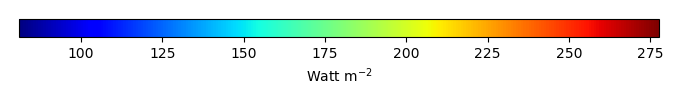MODEL MEANBIAS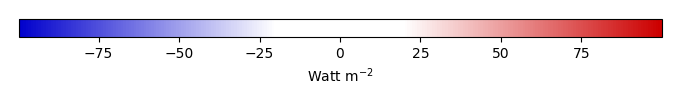BIAS SCORERMSE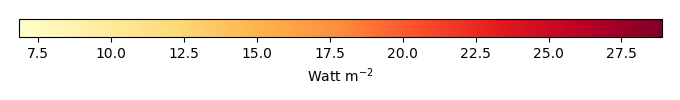RMSE SCOREBENCHMARK INTERANNUAL VARIABILITY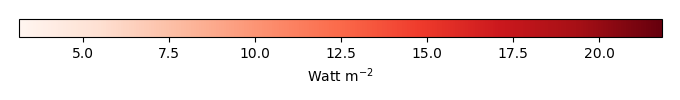MODEL INTERANNUAL VARIABILITYINTERANNUAL VARIABILITY SCOREBENCHMARK MAX MONTHMODEL MAX MONTHDIFFERENCE IN MAX MONTH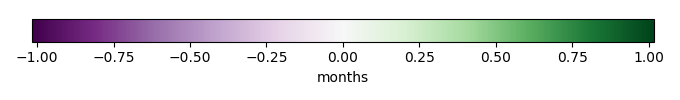SEASONAL CYCLE SCORESPATIAL TAYLOR DIAGRAMMODEL COLORS# Spatially integrated regional mean

MODEL COLORSREGIONAL MEANANNUAL CYCLEMONTHLY ANOMALYANNUAL CYCLE# All Models

BenchmarkCLM4CLM4.5CLM5# Data Information

creation_date: Mon Jun 30 23:16:59 PDT 2014

source_file: This product is generated from monthly 1 degree GEWEX SRB Radiation observations